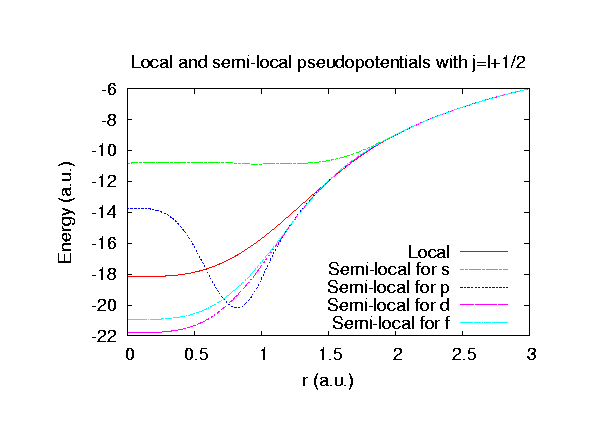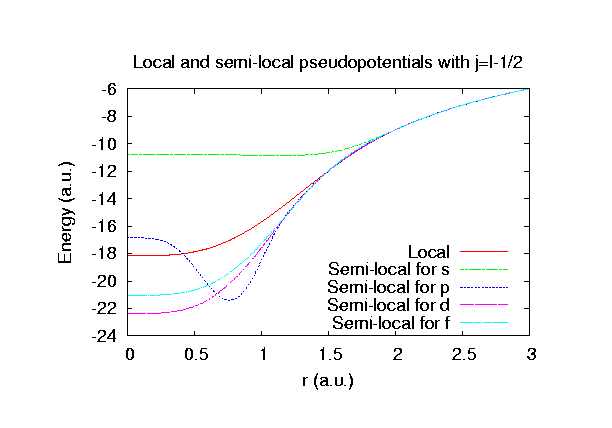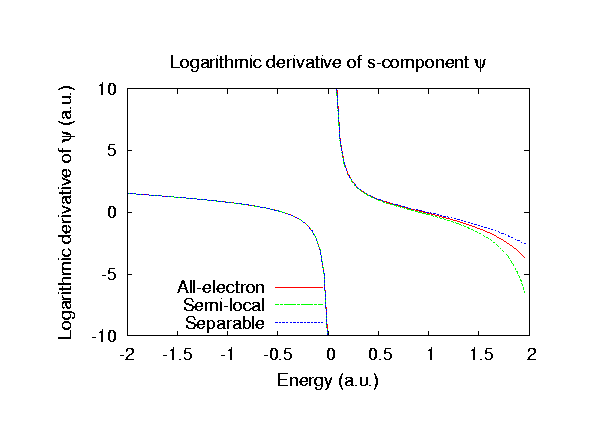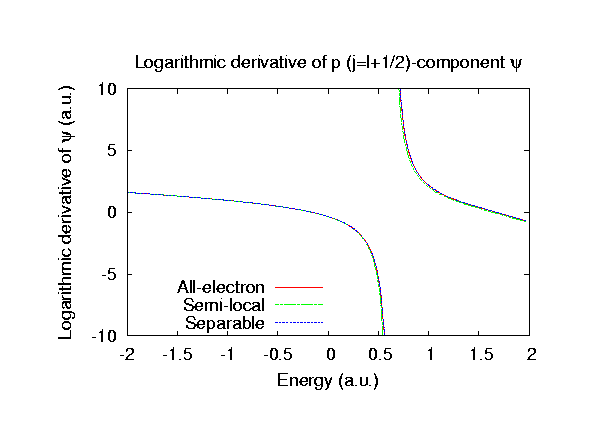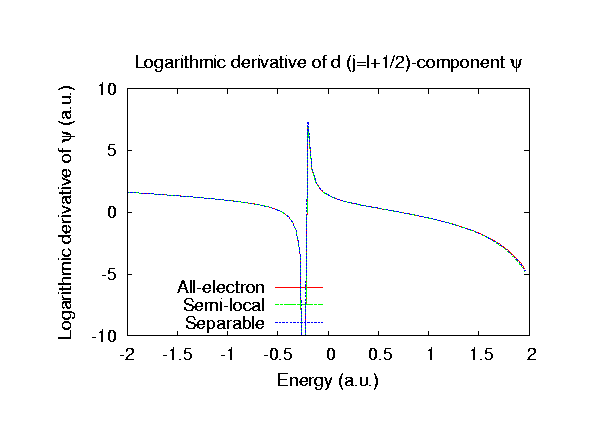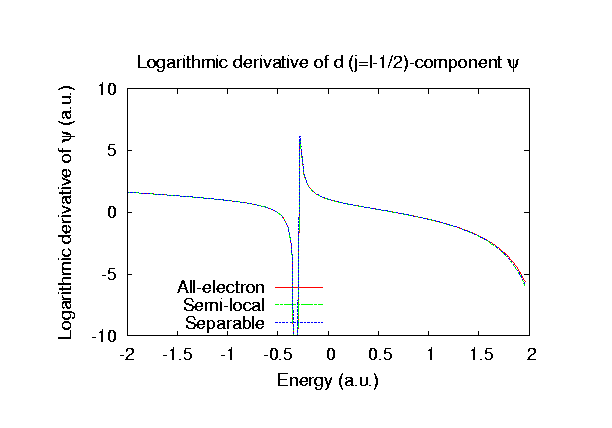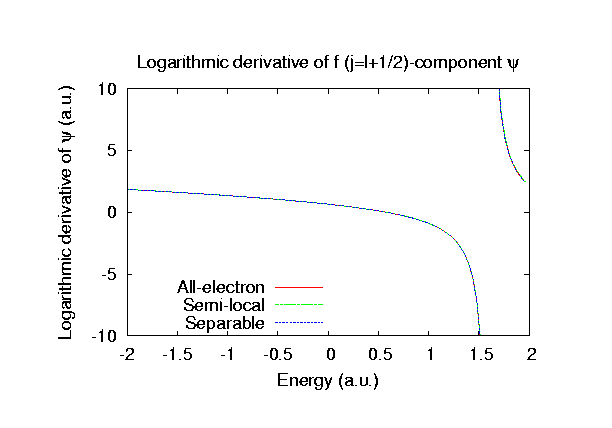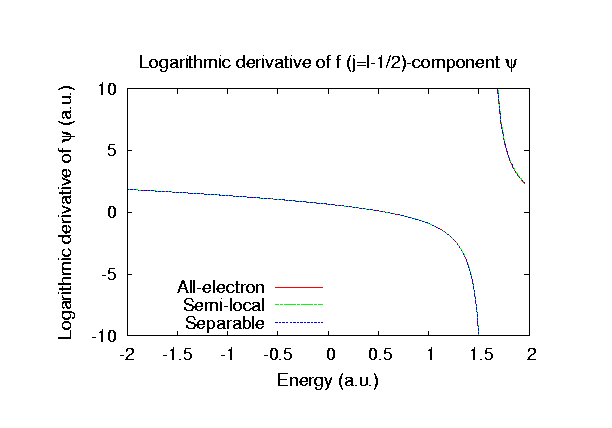### Fully relativistic pseudopotentials

Fully relativistic pseudopotentials generated by the MBK (PRB 47, 6728 (1993)) scheme within LDA (CA13) and GGA (PBE13) which contain a partial core correction and fully relativistic effects including spin-orbit coupling.

### Pseudo-atomic orbitals

The number below the symbol means a cutoff radius (a.u.) of the confinement potential. These file includes fifteen radial parts for each angular momentum quantum number l (=0,1,2,3,4). The basis functions were generated by variationally optimizing the corresponding primitive basis functions in the single atom and the FCC bulk. The input files used for the orbital optimization can be found at Hg_opt.dat and Hgfcc_opt.dat . Since Hg_CA13.vps and Hg_PBE13.vps include the 5p, 5d, and 6s (18 electrons) as the valence states, the minimal basis set is Hg*.*-s1p1d1. Our recommendation for the choice of cutoff radius of basis functions is that Hg8.0.pao is enough for bulks, but Hg10.0.pao or Hg12.0.pao is preferable for molecular systems.

### Benchmark calculations by the PBE13 pseudopotential with the various basis functions

(1) Calculation of the total energy as a function of lattice constant in the fcc structure, where the total energy is plotted relative to the minimum energy for each case. a0 and B0 are the equilibrium lattice constant and bulk modulus obtained by fitting to the Murnaghan equation of state. The difference between Hg8.0-s3p3d2f1 and Hg8.0-s4p3d3f2 in the total energy at the minimum point is 0.0043 eV/atom. An input file used for the OpenMX calculations can be found at Hgfcc-EvsV.dat . For comparison the result by the Wien2k code is also shown, where the calculation was performed by default setting in the Ver. 9.1 of Wien2k except for the use of RMT x KMAX of 12.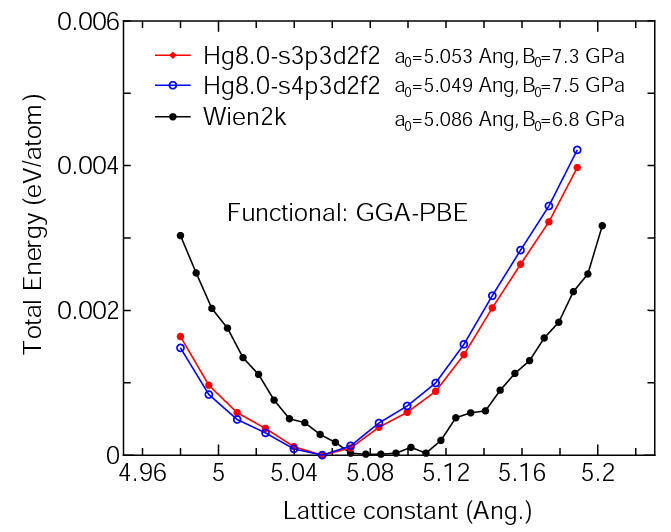(2) Calculations of the band dispersion in the fcc structure, where the non-spin polarized collinear calculation with the lattice constant of 4.920 Ang. was performed using Hg_PBE13.vps and Hg7.0-s2p3d2f1, and the origin of the energy is taken to be the Fermi level. The input file used for the OpenMX calculations can be found at Hgfcc-Band.dat . For comparison the result by the Wien2k code is also shown, where the calculation was performed by default setting in the Ver. 9.1 of Wien2k except for the use of RMT x KMAX of 12.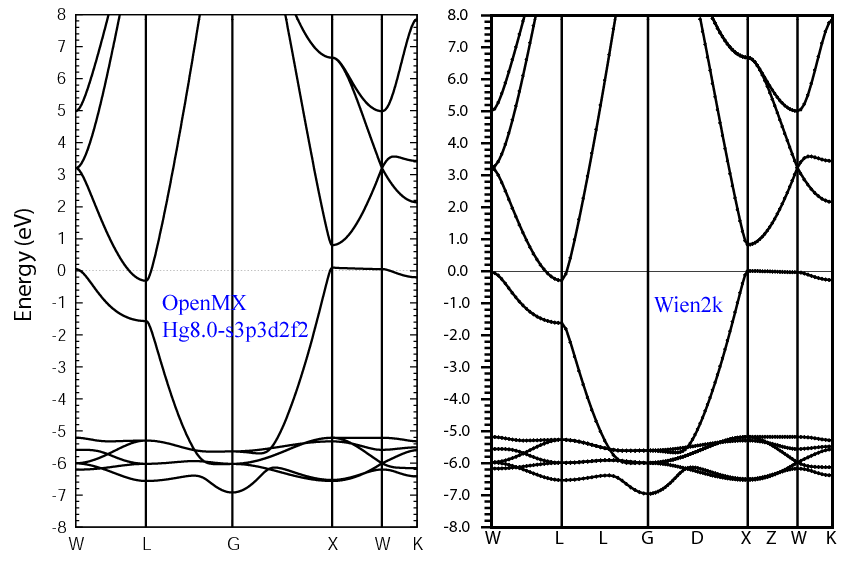### Supplementary information for the GGA (PBE13) pseudopotential